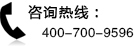### 12月CFA考试大纲：SS 2 Quantitative Methods Basic Concepts

201412CFA考试悄然而至，北京国际金融培训中心给大家带来总共18期的《201412CFA考试大纲》第一期，提前帮助大家对CFA考试有一个充分的认识。

201412CFA考试大纲：SS 2 Quantitative Methods Basic Concepts

Quantitative Methods Basic Concepts

This introductory study session presents the fundamentals of some quantitative techniques essential in financial analysis. These techniques are used throughout the CFA Program curriculum. This session introduces several tools of quantitative analysis: the time value of money, descriptive statistics, and probability.

Time value of money techniques are used throughout financial analysis. Time value of money calculations are the basic tools used to support corporate finance decisions and to estimate the fair value of fixed income, equity, and other types of securities or investments.

Descriptive statistics provide essential tools for describing and evaluating return and risk. Probability theory concepts are needed to understand investment decision-making under conditions of uncertainty.

Reading 5 The Time Value of Money Quantitative Methods for Investment Analysis, Second Edition, by Richard A. DeFusco, CFA, Dennis W. McLeavey, CFA, Jerald E. Pinto, CFA, and David E. Runkle, CFA

Reading 6 Discounted Cash Flow Applications Quantitative Methods for Investment Analysis, Second Edition, by Richard A. DeFusco, CFA, Dennis W. McLeavey, CFA, Jerald E. Pinto,CFA, and David E. Runkle, CFA

Reading 7 Statistical Concepts and Market Returns Quantitative Methods for Investment Analysis, Second Edition, by Richard A. DeFusco, CFA, Dennis W. McLeavey, CFA, Jerald E. Pinto, CFA, and David E. Runkle, CFA

Reading 8 Probability Concepts Quantitative Methods for Investment Analysis, Second Edition, by Richard A. DeFusco, CFA, Dennis W. McLeavey, CFA, Jerald E. Pinto, CFA, and David E. Runkle, CFA

LEARNING OUTCOMES

READING 5. THE TIME VALUE OF MONEY

The candidate should be able to:

a interpret interest rates as required rates of return, discount rates, or opportu?nity costs;

b explain an interest rate as the sum of a real risk-free rate, and premiums that compensate investors for bearing distinct types of risk;

c calculate and interpret the effective annual rate, given the stated annual interest rate and the frequency of compounding;

d solve time value of money problems for different frequencies of compounding;

e calculate and interpret the future value (FV) and present value (PV) of a single sum of money, an ordinary annuity, an annuity due, a perpetuity (PV only), and a series of unequal cash flows;

f demonstrate the use of a time line in modeling and solving time value of money problems.

READING 6. DISCOUNTED CASH FLOW APPLICATIONS

The candidate should be able to:

a calculate and interpret the net present value (NPV) and the internal rate of return (IRR) of an investment;

b contrast the NPV rule to the IRR rule, and identify problems associated with the IRR rule;

c calculate and interpret a holding period return (total return);

d calculate and compare the money-weighted and time-weighted rates of return of a portfolio and evaluate the performance of portfolios based on these measures;

e calculate and interpret the bank discount yield, holding period yield, effective annual yield, and money market yield for U.S. Treasury bills and other money market instruments;

f convert among holding period yields, money market yields, effective annual yields, and bond equivalent yields.

READING 7. STATISTICAL CONCEPTS AND MARKET RETURNS

The candidate should be able to:

a distinguish between descriptive statistics and inferential statistics, between a population and a sample, and among the types of measurement scales;

b define a parameter, a sample statistic, and a frequency distribution;

c calculate and interpret relative frequencies and cumulative relative frequencies, given a frequency distribution;

d describe the properties of a data set presented as a histogram or a frequency polygon;

e calculate and interpret measures of central tendency, including the population mean, sample mean, arithmetic mean, weighted average or mean, geometric mean, harmonic mean, median, and mode;

f calculate and interpret quartiles, quintiles, deciles, and percentiles;

g calculate and interpret 1) a range and a mean absolute deviation and 2) the variance and standard deviation of a population and of a sample;

h calculate and interpret the proportion of observations falling within a specified number of standard deviations of the mean using Chebyshevs inequality;

i calculate and interpret the coefficient of variation and the Sharpe ratio;

j explain skewness and the meaning of a positively or negatively skewed return distribution;

k describe the relative locations of the mean, median, and mode for a unimodal, nonsymmetrical distribution;

l explain measures of sample skewness and kurtosis;

m compare the use of arithmetic and geometric means when analyzing investment returns.

The candidate should be able to:

a define a random variable, an outcome, an event, mutually exclusive events, and exhaustive events;

b state the two defining properties of probability and distinguish among empiri?cal, subjective, and a priori probabilities;

c state the probability of an event in terms of odds for and against the event;

d distinguish between unconditional and conditional probabilities;

e explain the multiplication, addition, and total probability rules;

f calculate and interpret 1) the joint probability of two events, 2) the probability that at least one of two events will occur, given the probability of each and the joint probability of the two events, and 3) a joint probability of any number of independent events;

g distinguish between dependent and independent events;

h calculate and interpret an unconditional probability using the total probability rule;

i explain the use of conditional expectation in investment applications;

j explain the use of a tree diagram to represent an investment problem;

k calculate and interpret covariance and correlation;

l calculate and interpret the expected value, variance, and standard deviation of a random variable and of returns on a portfolio;

m calculate and interpret covariance given a joint probability function;

n calculate and interpret an updated probability using Bayes formula;

o identify the most appropriate method to solve a particular counting problem, and solve counting problems using factorial, combination, and permutation concepts.

本文出处：http://cfa.bjfinance.net/转载请注明

•北京CFA微信

•金程CFA微博